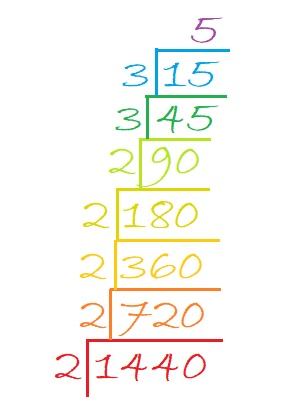# Taking the Mystery out of 1440

The number 1440 has thirty-six factors. That’s a lot! The only number smaller than it with that many factors is 1260. Why do they have so many?

Would solving this Mystery Level puzzle be an easier mystery to solve?Print the puzzles or type the solution in this excel file: 10 Factors 1432-1442

• 1440 is a composite number.
• Prime factorization: 1440 = 2 × 2 × 2 × 2 × 2 × 3 × 3 × 5, which can be written 1440 = 2⁵ × 3² × 5
• 1440 has at least one exponent greater than 1 in its prime factorization so √1440 can be simplified. Taking the factor pair from the factor pair table below with the largest square number factor, we get √1440 = (√144)(√10) = 12√10
• The exponents in the prime factorization are 5,2, and 1. Adding one to each exponent and multiplying we get (5 + 1)(2 + 1)(1 + 1) = 6 × 3 × 2 = 36. Therefore 1440 has exactly 36 factors.
• The factors of 1440 are outlined with their factor pair partners in the graphic below.There you have it. The exponents of the prime factorization determine the number of factors a number has, and (5 + 1)(2 + 1)(1 + 1) = 6 × 3 × 2 = 36. How do we find its prime factorization? We can divide it over and over by the smallest prime number factor until we make an easy-to-read and very delicious cake!Or you could make a factor tree such as the one below. Then rake up the prime number factors afterward and hope that you see them all:I prefer cake to yard work any day!

As I mentioned before, 1440 is NOT the smallest number with 36 factors:
Because (2 + 1)(2 + 1)(1 + 1)(1 + 1) = 3 × 3 × 2 × 2 = 36 also, we get
2²× 3²× 5 × 7 = 1260, which just happens to be smaller than 1440, so 1260 gets the smallest-number-with-36-factors prize. 1440 is the SECOND smallest number with 36 factors.

1440 is the sum of the interior angles of a decagon. Why?
Because 180(10-2) = 180(8) = 1440
So what? 1260 is the sum of the interior angles of a nonagon.

1440 is the hypotenuse of only one Pythagorean triple:
864-1152-1440 which is (3-4-5) times 288
Yeah? 1260 is the hypotenuse of only one Pythagorean triple as well.

Look, 1440 is not second best because it has this one other claim to fame:
From OEIS.org, we learn that 1440 deserves a lot of exclamation points since
1440 = 2!3!5!

There you have it. We’ve taken the mystery out of the number 1440, AND it is a fabulous number!

This site uses Akismet to reduce spam. Learn how your comment data is processed.